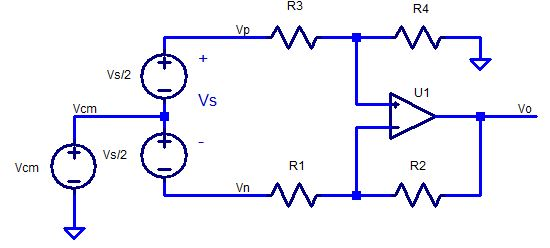## Op Amp Differential Amplifier

Enter the following: Vs, Vcm and R1 - R4. Then click on "Get Gains, Vo".

The Differential Signal Gain (Kdiff) should be R2/R1 if R4/R3=R2/R1.

Ideally, Vo due to the Common Mode Level should be 0V (Kcm=0).
But it's difficult to acheive with resistor tolerances.
Try setting R1=10100 (1% error), R3=10000, R2=R4=20000, vs=0 and vcm=5.
How much unwanted common-mode signal gets through to Vo?Vs Signal (vs = Vp-Vn) Vcm Common Mode (Signal Offset) R1 Gain Resistors R2 R3 R4 Kp=Vo/Vp Pos Gain: Kp=R4/(R3+R4)*(R2+R1)/R2 Kn=Vo/Vn Neg Gain: Kn=-R2/R1 Kdiff Differential Gain: Kdiff = Vo/Vs = Kp/2 - Kn/2 Kcm Common Mode Gain: Kcm = Vo/Vcm = Kp + Kn Vp (V) Vp = Vcm + Vs/2 Vn (V) Vn = Vcm - Vs/2 Vo (V) Vo = Kp*Vp + Kn*Vn

To run or modify on your PC, download a simple version of this file with image (*.zip).
For a quick tour of some JavaScript / HTML basics, check out Inside the Code.

### JavaScript

This code features one function call associated with the "Get_R2, Kn and fc" button. Here's the code.

```//////////////////////////////////////////////
// calc Diff Amp
//////////////////////////////////////////////
function get_DiffAmp() {

// get values directly from form
var vs=document.myForm.vs.value;
var vcm=document.myForm.vcm.value;
var R1=document.myForm.R1.value;
var R2=document.myForm.R2.value;
var R3=document.myForm.R3.value;
var R4=document.myForm.R4.value;

// convert text to number
R1=Number(R1);
R2=Number(R2);
R3=Number(R3);
R4=Number(R4);
vs=Number(vs);
vcm=Number(vcm);

var Kp, Kn, vp, vn, Kdiff, Kcm, vo;

// calc gains and output
Kn = -R2/R1;
Kp = R4/(R3+R4)*(R1+R2)/R1;
vp = vcm + 1/2*vs;
vn = vcm - 1/2*vs;
Kcm = Kp+Kn;
Kdiff = Kp/2-Kn/2;
vo = vp*Kp + vn*Kn;

// place in text box
document.myForm.Kp.value = (Kp).toPrecision(6);
document.myForm.Kn.value = (Kn).toPrecision(6);
document.myForm.vp.value = (vp).toPrecision(6);
document.myForm.vn.value = (vn).toPrecision(6);
document.myForm.Kcm.value = (Kcm).toPrecision(6);
document.myForm.Kdiff.value = (Kdiff).toPrecision(6);
document.myForm.vo.value = (vo).toPrecision(3);
```

The two radio buttons, both named "config", create an array of elements config and config for the buttons named "Non-Inverting" and "Inverting". You can use the array to determine which button was checked as shown in the code above.

### HTML

Here's the HTML for the radio butons to select the amplifier configuration. Note that both are named "config". You can set which button is initially selected by including "checked" in the input line.